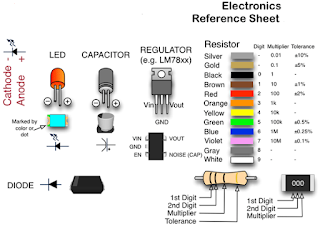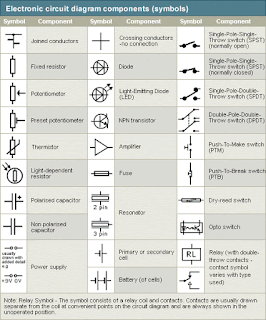## Tuesday 16 June 2015

### Electrical Technology

In electrical engineering, we are often interested in communicating orComponent Image
transferring energy from one point to another. To do this requires an
interconnection of electrical devices. Such interconnection is referred to
as an electric circuit, and each component of the circuit is known as an
element.
Circuit Elements:
There are 2 types of elements found in electrical
circuits.
1) Active elements (is capable of generating energy), e.g., generators,batteries, and operational ampli ers (Op-amp).

2) Passive element, e.g., resistors, capacitors and inductors.

The most important active elements are voltage and current
sources:
(a) Voltage source provides the circuit with a speci ed voltage (e.g. a 1.5V battery)
(b) Current source provides the circuit with a speci ed current (e.g. a 1A current source).There are two fundamental laws that govern electric circuits (Ohm's law and Kirchho 's laws)

Ohm's Law: The voltage v across a resistor is directly propor-tional to the current i flowing through the resistor.
v =iR
where R = resistance of the resistor, denoting its ability to resist the flow of electric current. The resistance is measured in ohms .
Kirchho 's Laws: Ohm's law coupled with Kirchho 's two laws gives a su cient, powerfulset of tools for analyzing a large variety of electric circuits.
1. Kirchho 's current law (KCL): the algebraic sum of current entering a node (or a closed boundary) is zero.KCL is based on the law of conservation of charge.
2.A Kirchho 's voltage law (KVL): the algebraic sum of all voltages around a closed path (or loop) is zero.KVL is based on the law of conservation of energy.